# php tutorial - PHP Arrays - php programming - learn php - php code - php script

## Arrays :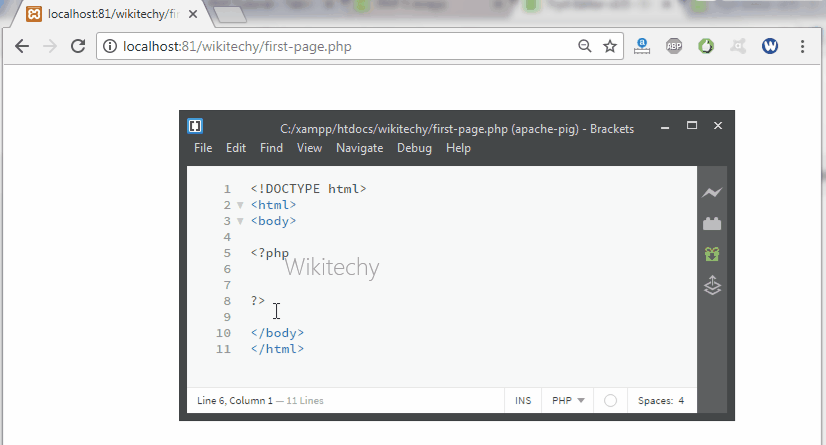Learn PHP - PHP tutorial - Array - PHP examples - PHP programs

• An array is a data structure that is used for storing more than one value in a single variable.
• In php using array () function we can create an “array”.
• The array values are defined in numbers (or) character.
• In array the values are separated by commas (,).

## php programming Syntax :

• There are three types of arrays used in php they are :
1. Indexed arrays / Numerical arrays - An array with a numeric index
2. Associative arrays - An array where each ID key is associated with a value
3. Multidimensional arrays - An array containing one or more arrays

## Indexed Arrays :

• In php generally the array index value is assigned automatically starting with Zero (0).
• A numeric array stores each array element with a numeric index.
• There are two methods to create a numeric array.
• In the following example the index is automatically assigned (the index starts at 0):
•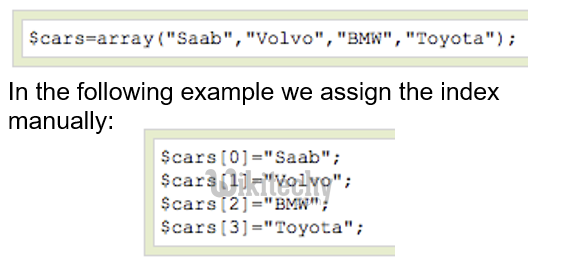• In the following example you access the variable values by referring to the array name and index:
•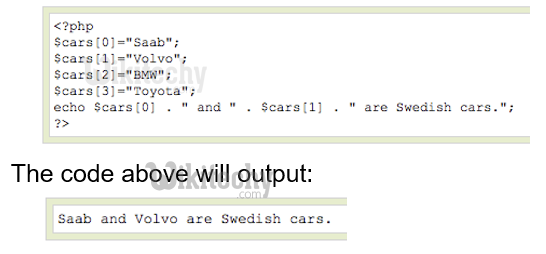## Associative Arrays :

• An associative array is defined as name with their key value.
• With an associative array, each ID key is associated with a value.
• When storing data about specific named values, a numerical array is not always the best way to do it.
• With associative arrays we can use the values as keys and assign values to them.
• In this example we use an array to assign ages to the different persons:
•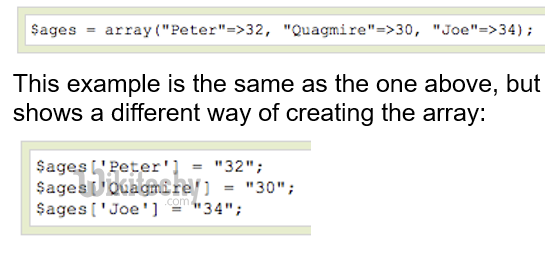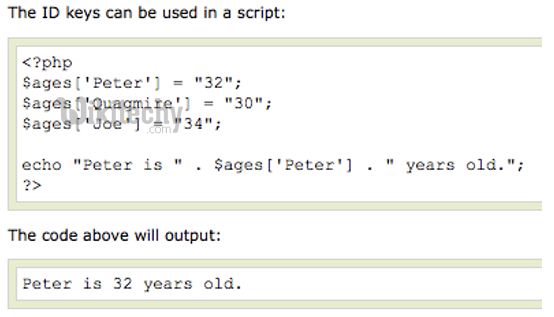## Multidimensional Arrays :

• A multidimensional array contains one are more arrays in a single variable.